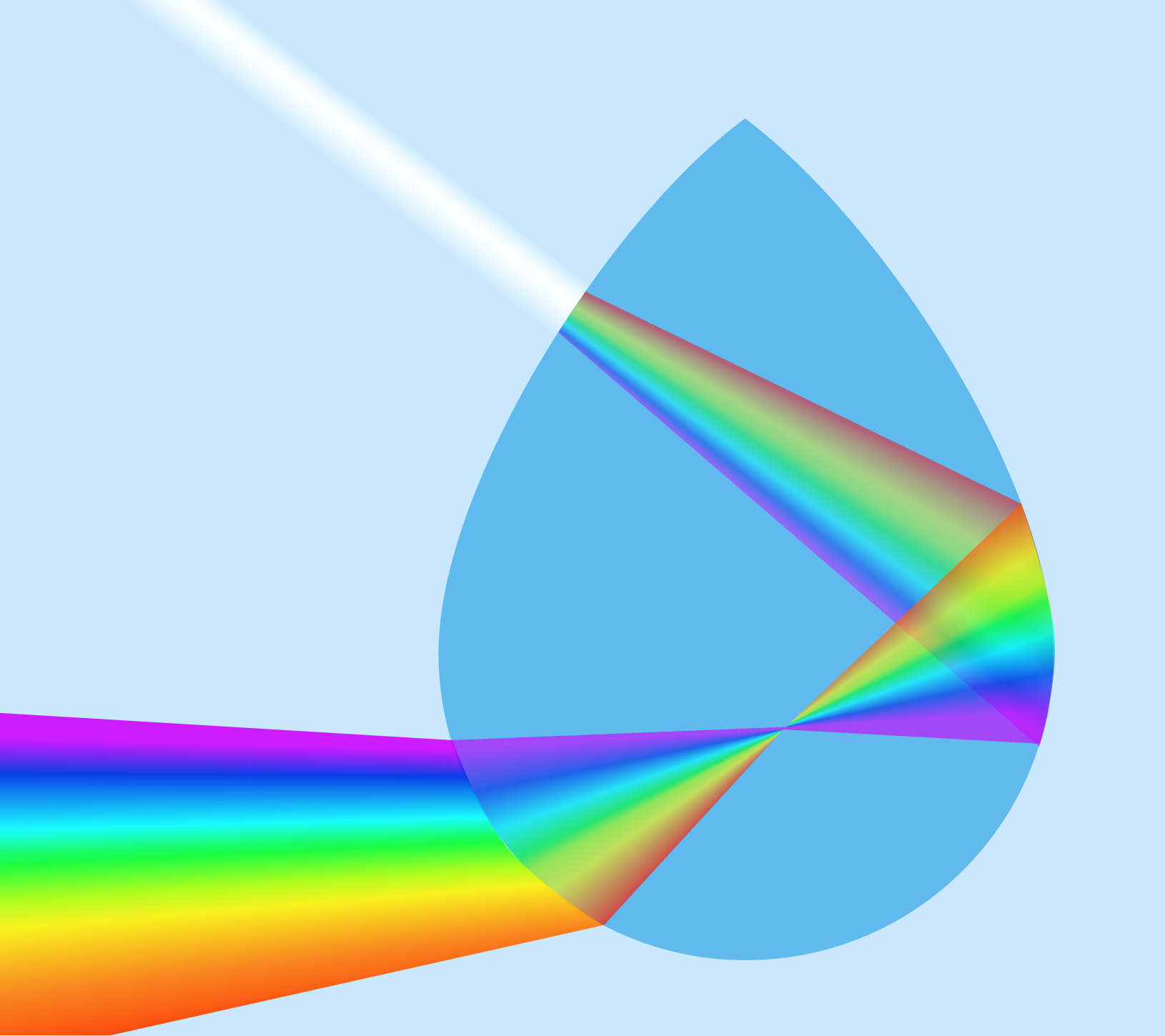# Problems of the Week

Contribute a problem

# 2018-08-27 Basic

\begin{aligned} \color{#D61F06}1\times8+1& =9 \\ \color{#3D99F6}12\times8+2 & =98 \\ \color{#EC7300}123\times8+3 & =987 \end{aligned}

It seems like there's a pattern here, but is the statement below also true? $123456789\times8+9 = 987654321$

If $A^B = 8^2,$ is it true that $A\times B$ must equal 16?An artist's rendering of refraction and reflection in a water droplet.

When it rains on a sunny day, sometimes we see rainbows—circular arcs of every color from red to violet. When sunlight hits a water droplet, the light bends and is reflected at a specific angle. Different colors of light bend differently, so the droplets split up sunlight into a band of colors.

If the conditions are right, which direction should you face to most likely see a rainbow?

How many different ways can you write 1001 as the sum of two primes?

Akshatha and Dev both take half an hour to finish their lunches. Each arrives at the canteen at a random time between 1:00 and 2:00 pm.

Is it more likely that they are both in the canteen at the same time for some amount of time than not?

×